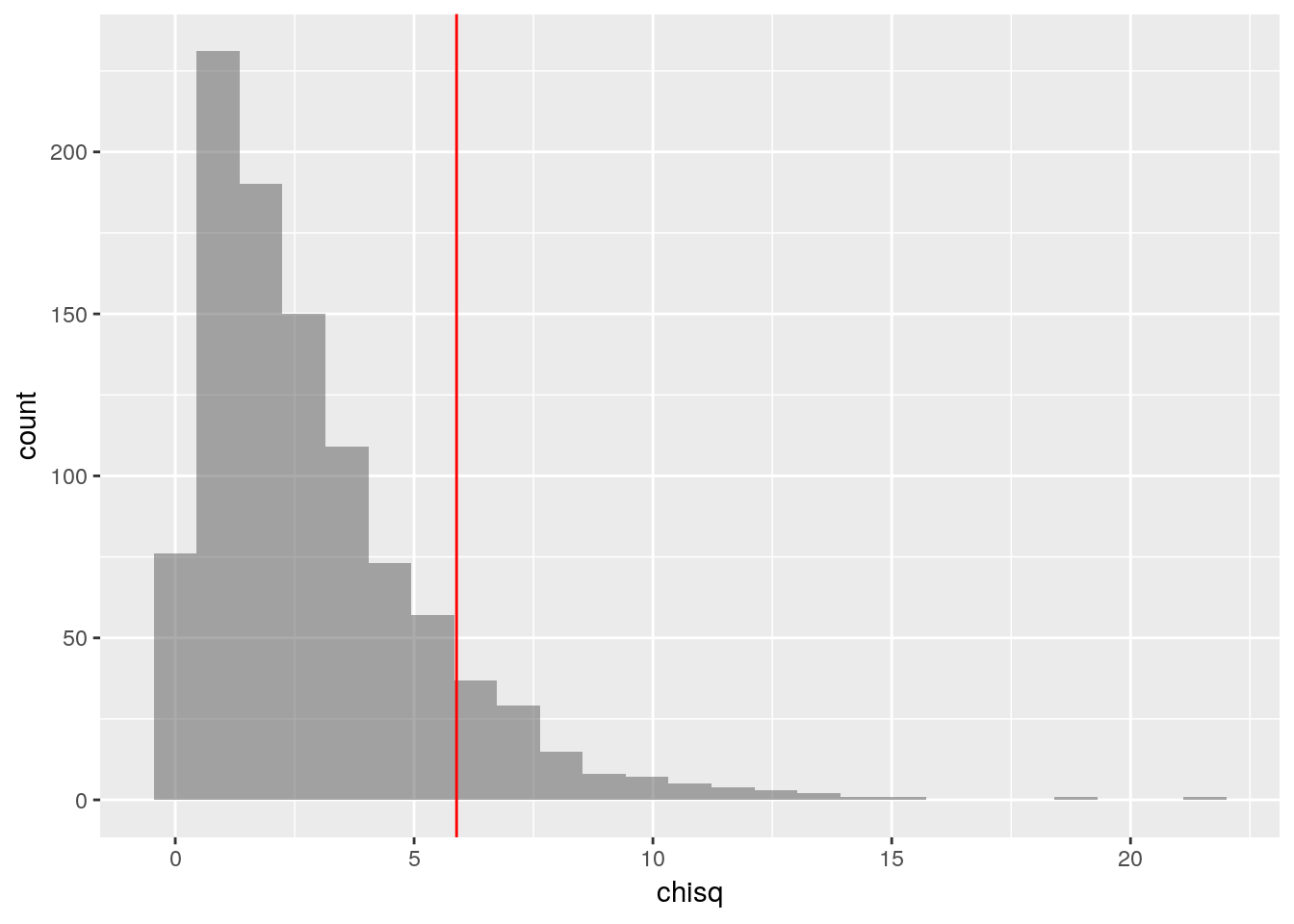# Rows in tidyverse again

Jan Hlavacek · 2019/04/30 · 4 minute read

In the last post I complained that there is no way to apply a formula to rows of a data frame so that the formula will consume the rows as lists or vectors. Turns out I was wrong, and there is a very easy way to do it!

Not only that, but I was actually really close to it, and if I was just reading the relevant documentation bit more carefully…

As I wrote in that post, this works:

selections %>%
mutate(chisq = pmap_dbl(., ~sum((c(..1, ..2, ..3, ..4) -
expected)^2/expected))) %>%
head()

I was suggesting that perhaps something like

pmap_dbl(selections, ~sum((.row - expected)^2/expected))

would be nice to have. While experimenting, among other things I tries this:

pmap_dbl(selections, ~sum((... - expected)^2/expected))

which of course does not work, and I am not sure why I thought it would. The correct way, of course, is

pmap_dbl(selections, ~sum((c(...) - expected)^2/expected))

The ... is, in this case, equivalent to ..1, ..2, ..3, ..4. Since I need c(..1, ..2, ..3, ..4), I have to use c(...).

## The simulation again

Anyway, let’s do the whole simulation from the previous post again:

library(tidyverse)
library(mosaic)

First enter the given information:

labels <- fct_inorder(c("White", "Black", "Hispanic", "Other"), ordered=TRUE)

percentages <- c(72, 7, 12, 9)
names(percentages) <- labels

observed <- c(205, 26, 25, 19)
names(observed) <- labels

Calculate the expected frequencies and the observed $$\chi^2$$ score:

expected <- 275*percentages/100

observed_chi_square <- sum((observed - expected)^2/expected)
observed_chi_square
##  5.88961

Repeatedly sample, with replacement, 275 pieces of paper from a bag representing the population, tally each sample, and record the frequencies in a data set:

bag <- rep(labels, percentages)
do(1000) * tally(sample(bag, 275, replace=TRUE)) -> selections
glimpse(selections)
## Observations: 1,000
## Variables: 4
## $White <int> 200, 187, 198, 196, 197, 200, 193, 187, 200, 196, 208, … ##$ Black    <int> 20, 19, 23, 23, 17, 20, 27, 17, 17, 20, 16, 25, 11, 19,…
## $Hispanic <int> 31, 36, 32, 34, 35, 30, 35, 38, 35, 36, 29, 39, 44, 30,… ##$ Other    <int> 24, 33, 22, 22, 26, 25, 20, 33, 23, 23, 22, 24, 24, 20,…

Add a new column to the data set, with the $$\chi^2$$ scores of the simulated samples:

selections %>%
mutate(chisq = pmap_dbl(., ~sum((c(...) - expected)^2/expected))) ->
selections_with_chisq

This is what we have now:

head(selections_with_chisq)
##   White Black Hispanic Other     chisq
## 1   200    20       31    24 0.1933622
## 2   187    19       36    33 3.6370851
## 3   198    23       32    22 1.0663781
## 4   196    23       34    22 1.0865801
## 5   197    17       35    26 0.4523810
## 6   200    20       30    25 0.3246753

Plot the $$\chi^2$$ scores of all the simulated samples, and mark the observed $$\chi^2$$ on the plot with a vertical line.

gf_histogram(~chisq, data = selections_with_chisq) %>%
gf_vline(xintercept = observed_chi_square, color="red")How many of the 1000 simulated samples had a $$\chi^2$$ score greater than or equal to the observed $$\chi^2$$ score?

count(~(chisq >= observed_chi_square), data = selections_with_chisq)
## n_TRUE
##    112

## Possible simplification

I am pretty happy with this. The only thing that I think can still confuse R beginners is the use of . as the first argument of pmap_dbl. I usually do few examples of tidyverse pipelines at the beginning of the semester, and usually one or two of those use this somewhere, but I don’t think most students will remember those at this point. Since we do not actually need to preserve the original columns (the only reason I preserved them was to make the data set look just like a worksheet they filled in while figuring out the whole idea of $$\chi^2$$ scores), we can do this instead:

selections %>%
pmap_df(~list(chisq = sum((c(...) - expected)^2/expected))) -> chisquares

and then replace selections_with_chisq by chisquares when making the histogram and calculating the p-value. I am not sure if this really is that much simpler, with the list, and pmap_df not being one of the basic dplyr verbs we learned at the beginning of the semester…

Edit this page on GitHub.